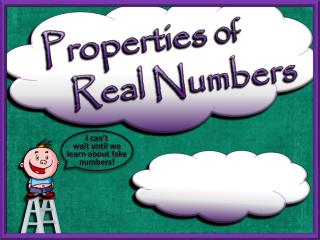DownloadDownload PresentationProperties 0f Real Numbers

# Properties 0f Real Numbers

Download Presentation## Properties 0f Real Numbers

- - - - - - - - - - - - - - - - - - - - - - - - - - - E N D - - - - - - - - - - - - - - - - - - - - - - - - - - -
##### Presentation Transcript

1. Properties 0f Real Numbers I can’t wait until we learn about fake numbers!

2. Properties 0f Real Numbers The properties of real numbers help us simplify math expressions and help us better understand the concepts of algebra.

3. Commutative Property of Addition a + b = b + a Example: 7 + 3 = 3 + 7 Two real numbers can be added in either order to achieve the same sum. Does this work with subtraction? Why or why not?

4. Commutative Property of Multiplication a x b = b x a Example: 3 x 7 = 7 x 3 Two real numbers can be multiplied in either order to achieve the same product. Does this work with division? Why or why not?

5. Associative Property of Addition (a + b) + c = a + (b + c) Example: (29 + 13) + 7 = 29 + (13 + 7) When three real numbers are added, it makes no difference which are added first. Notice how adding the 13 + 7 first makes completing the problem easier mentally.

6. Associative Property of Multiplication (a xb) xc = a x (b xc) Example:(6 x 4) x 5 = 6 x (4 x 5) When three real numbers are multiplied, it makes no difference which are multiplied first. Notice how multiplying the 4 and 5 first makes completing the problem easier.

7. Additive Identity Property a + 0 = a Example: 9 + 0 = 9 The sum of zero and a real number equals the number itself. Memory note: When you add zero to a number, that number will always keep its identity.

8. Multiplicative Identity Property ax 1= a Example:8x1 = 8 The product of one and a number equals the number itself. Memory note: When you multiply any number by one, that number will keep its identity.

9. Properties 0f Real Numbers TIME OUT!!! My brain’s about to explode with all of this crazy property stuff! What’s wrong? Hang in there, Sparky! We only have a few more.

10. Distributive Property a(b + c) = ab + acora(b – c) = ab – ac Example:2(3 + 4) = (2x 3) + (2x 4) or 2(3 - 4) = (2x 3) - (2x 4) Distributive Property is the sum or difference of two expanded products.

11. Additive Inverse Property a + (-a) = 0 Example: 3 + (-3) = 0 The sum of a real number and its opposite is zero.

12. Multiplicative Inverse

13. You are now ONE with the properties of real numbers. Sparky’s fixin’ to become one with a triple decker, chili- fire bean burrito! Go forth and use them wisely! Use them confidently! And use them well, my friends! My job here is done!